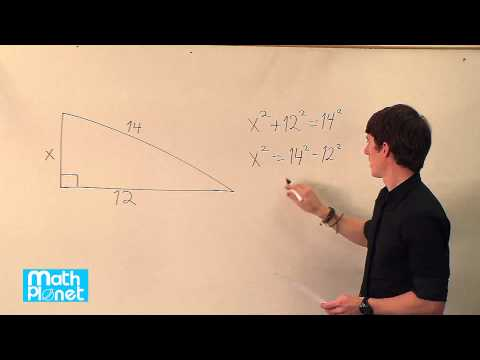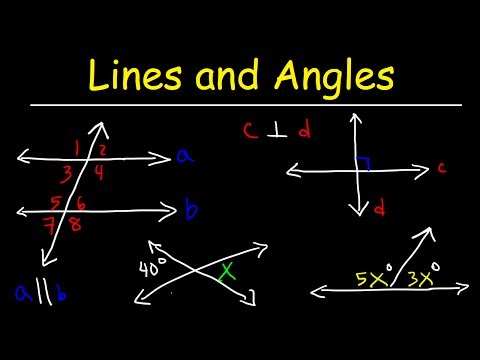# Proof: Alternate Exterior Angles Converse

##By Mathispower4u

Proof: Alternate Exterior Angles Converse# Another Pythagorean theorem proof | Right triangles and trigonometry | Geometry | Khan Academy

##By Khan Academy

Khan Academy presents Another Pythagorean Theorem Proof, an educational video resource on math.# The converse of the Pythagorean theorem and special triangles

##By MathPlanetVideos

Find the value of x in the right triangle.# Pythagorean theorem 3 | Right triangles and trigonometry | Geometry | Khan Academy

##By Khan Academy

This three-minute video is from Sal Khan about using the Pythagorean Theorem to solve a word problem: A carpet measures 7 feet long and has a diagonal measurement of square root of 74 feet. Find the width of the carpet Mr. Khan uses computer software for demonstration. The screen is a little 'busy' so the viewer may want to open the screen to 'full screen' to see all more clearly.# Proof of Pythagorean Theorem using similar triangles

##By Matt Stryker

Proof of Pythagorean Theorem using similar triangles# The Pythagorean theorem intro | Right triangles and trigonometry | Geometry | Khan Academy

##By Khan Academy

Watch this video to review right triangles and the Pythagorean Theorem view an example of using the Pythagorean Theorem to find the length of a hypotenuse (the longest side of a right triangle) view an example of using the Pythagorean Theorem to find the length of a leg of a right triangle# Parallel and Perpendicular Lines, Transversals, Alternate Interior Angles, Alternate Exterior Angles

##By The Organic Chemistry Tutor

parallel and perpendicular lines in addition to transversals and all the angles that are formed by it such as alternate interior and exterior angles.# Pythagorean theorem 1 | Right triangles and trigonometry | Geometry | Khan Academy

##By Khan Academy

This is a Khan Academy video about solving a word problem involving the need to find the length of a rope that attaches a mast of a boat with the deck of the boat.# Pythagorean theorem 2 | Right triangles and trigonometry | Geometry | Khan Academy

##By Khan Academy

In this video, Sal Khan continues discussing the Pythagorean Theorem to solve for the third side of the triangle Mr. Khan uses computer software for demonstration. The screen is a little 'busy' so the viewer may want to open the screen to 'full screen' to see all more clearly.# Proof: Right triangles inscribed in circles | High School Math | Khan Academy

##By Khan Academy

Proof showing that a triangle inscribed in a circle having a diameter as one side is a right triangle. All Khan Academy content is available for free at www.khanacademy.org